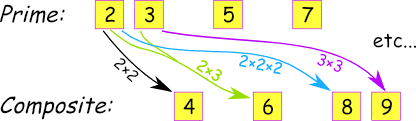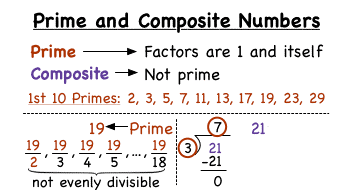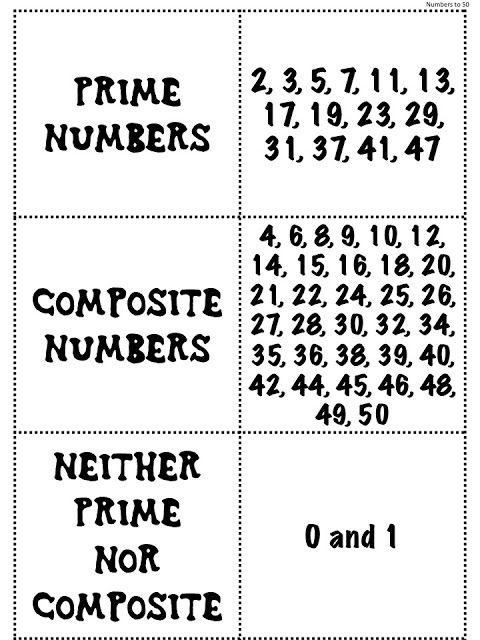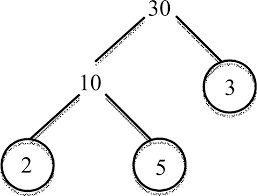Mathematics

# What are Composite Numbers?

420 views

 1 Definition and Types of Composite Numbers 2 Determine if a number is composite or not 3 List of Composite Numbers 4 Prime Factorization 5 Is there a final Prime? 6 Frequently Asked Questions (FAQs)

18th September 20

In Mathematics, Composite numbers are the numbers that have more than two factors, unlike prime numbers, which have only two factors, i.e., one and the number itself. Such numbers with more than two factors also are called composites.

Natural numbers greater than one that aren't prime are called composites because they're divisible by more than two numbers. The numbers $$0$$ and $$1$$ are neither prime nor composite, and therefore $$2$$ is the first Prime Number and $$4$$ the first composite number.All the natural numbers which aren't prime numbers are composite as they will be divided by more than two numbers. For instance, $$6$$ is composite because it's divisible by $$1, 2, 3,$$ and even $$6,$$ such as:

\begin{align}6÷1 &= 6\\\\6÷2 &= 3\\\\6÷3 &= 2\\\\6÷6 &= 1\end{align}

## Definition and Types of Composite Numbers

The integers which may be generated by multiplying the $$2$$ smallest positive integers and containing a minimum of one divisor aside from number ‘$$1$$’ and itself are understood as composite numbers. These numbers always have more than two factors.

### Definitions

1. A prime number is defined as the number that has only two factors: Number $$1$$ and itself.

2. A composite number has more than two factors.

3. Some numbers like number $$1$$ and $$0$$ can neither be called prime nor composite.

### Types of Composite Numbers:

There are two ways of classifying composite Numbers:

Here are two types of composite Numbers:

1. Odd Composite Numbers or Composite Odd Numbers

2. Even Composite Numbers or Composite Even Numbers

1. Odd Composite Numbers

All the odd integers that are not prime integers are odd composite numbers.

Examples of composite odd numbers are $$9, 15, 21,25, 27, 31,$$etc.

E.g.Consider the numbers $$1, 2, 3, 4, 9, 10, 11,12,$$ and $$15 .$$

The odd composite numbers here are $$9$$ and $$15$$ because those two numbers have the odd divisors and satisfy the composite condition.

2. Even Composite Numbers

All the even integers which are not prime integers are even composite numbers.

Samples of even composite numbers are $$4, 6, 8, 10, 12, 14, 16,$$ etc.

E.g. Consider the numbers $$1, 2, 3, 4, 9, 10, 11,12,$$ and $$15$$ again .

Here are the two numbers that have the odd divisors and satisfy the even composite conditions are 4, 10, and 12.

## How to Determine if a number is Composite or not?

The procedures to seek out whether a given number is prime or composite:

1. One way to classify composite numbers is by counting the number of prime factors.
1. Another way to classify composite numbers is by counting the number of divisors.

All composite numbers have a minimum of three divisors.

A number could also be a positive integer that can be formed by multiplying two smaller positive integers. Equivalently, it is a positive integer that features a minimum of 1 divisor apart from 1 and itself.

Every positive integer is composite, prime, or unit 1, therefore the composite numbers are precisely the numbers that are not prime and not a unit.Example:

Find out if $$14$$ may be a composite number.

Let us find the factors for $$14.$$

\begin{align}14÷1 = 14\\\\14÷2 = 7\\\\14÷7 = 2\\\\14÷14 = 1 \end{align}

As we will see, the factors of $$14$$ are $$1, 2, 7$$ and $$14,$$ so it's a composite number.

 Example :

Here are the numbers from 1 to 14. We can easily find out which number is composite and which number is a prime number from this table.

No.

Fact Prime/Composite
1 (1 isn't prime or composite)
2 1, 2 Prime
3 1, 3 Prime
4 1, 2, 4 Composite
5 1, 5 Prime
6 1, 2, 3, 6 Composite
7 1, 7 Prime
8 1, 2, 4, 8  Composite
9 1, 3, 9 Composite
10 1, 2, 5, 10 Composite
11 1, 11  Prime
12 1, 2, 3, 4, 6, 12  Composite
13 1, 13 Prime
14 1, 2, 7, 14 Composite

## List of Composite Numbers

The list of prime and composite numbers up to 50 are:

The positive integers having quite two factors are composite numbers. For example, $$4, 6, 8, 9,$$ and $$10$$ are the primary few composite numbers.## Prime Factorization

Prime factorization of a number is defined as the process of expressing that number as the product of just its prime factors.

When numbers are multiplied, they're called factors.

Since

$$30 = 2 \times15,$$

We say that $$2$$ and $$15$$ are factors of $$30.$$ $$2$$ may now be a prime divisor but $$15$$ isn't. However, $$15 = 3 × 5.$$ Therefore, we will express $$30$$ as a product of prime factors only:

$$30 = 2 \times 3 \times 5.$$

"$$2 \times 3 \times 5$$" is named the prime factorization of $$30.$$ And it's unique. That is, aside from the order of the factors:All numbers are often uniquely factored as a product of prime numbers only.

Note: We could have found those self-same factors by factoring $$30$$ in any way. for instance,

$$30 = 5 × 6.$$

And since $$6 = 2 \times 3,$$

$$30 = 5 \times 2 \times 3.$$

Apart from the order, we've found an equivalent prime factor.

## Is there a final prime?

The larger the numbers get, the greater the likelihood that they're going to have a divisor and be composite. So there might be a final prime.

Now, if there would have been a final prime, then we could imagine an inventory that contains every prime up to and including the last one.

1. Assume there are a finite number of primes, $$\text{Pn}$$ being the largest one.
2. Consider a number $$\text{N=P1*P2*....*Pn + 1}$$
3. By Construction, $$\rm{N}$$ is not divisible by any of $$\text{P1, P2,...., Pn}$$
4. Hence, it is either prime or divisible by a prime number greater than Pn which contradicts our assumption

We can now prove that there's no such list, which is to mention, there's no last prime.

-Written by Medha Sharma, Cuemath Teacher

## 1. Is 0 a composite number?

The condition of a prime number is that the number has exactly two factors, one is 1 and the other is the number itself and the condition of the Composite Number is that number has more than two factors, other than 1 and the number itself.

Since 1 can not be divided by any other number than itself and any number can not be the factor of 1. Neither 1(One) follow the condition of Prime Numbers nor the condition of Composite Numbers.

That is proved that Number 1 is neither a prime number nor a Composite Number.

## 2. Is 1 a composite number?

The condition of a prime number is that the number has exactly two factors, one is 1 and the other is the number itself and the condition of the Composite Number is that number has more than two factors, other than 1 and the number itself.

Since 1 can not be divided by any other number than itself and any number can not be the factor of 1. Neither 1(One) follow the condition of Prime Numbers nor the condition of Composite Numbers.

That is proved that Number 1 is neither a prime number nor a Composite Number.

## 3. Is 2 a composite number?

Integer 2 has no more than two factors, other than 1 and the number itself so that does not follow prime conditions.

Number two(2)has exactly two factors, one is 1, and the other is the number itself that satisfies the prime number condition.

So 2 is not a composite number.

## 4. Can you define the composite numbers from 1 to 100?

 4 6 8 9 10 12 14 15 16 18 20 21 22 24 25 26 27 28 30 32 33 34 35 36 38 39 40 42 44 45 46 48 49 50 51 52 54 55 56 57 58 59 60 62 63 64 65 66 68 69 70 72 74 75 76 78 80 81 82 84 85 86 87 88 90 91 92 94 96 98 99 100

GIVE YOUR CHILD THE CUEMATH EDGE
Access Personalised Math learning through interactive worksheets, gamified concepts and grade-wise courses